Next: Spin Greater Than One-Half Up: Spin Angular Momentum Previous: Spinor Rotation Matrices

# Factorization of Spinor-Wavefunctions

In the absence of spin, the Hamiltonian can be written as some function of the position and momentum operators. Using the Schrödinger representation, in which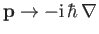, the energy eigenvalue problem,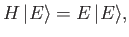(5.105)

can be transformed into a partial differential equation for the wavefunction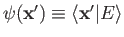. This function specifies the probability density for observing the particle at a given position,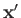. In general, we find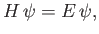(5.106)

where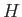is now a partial differential operator. The boundary conditions (for a bound state) are obtained from the normalization constraint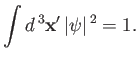(5.107)

This is all very familiar. However, we now know how to generalize this scheme to deal with a spin one-half particle. Instead of representing the state of the particle by a single wavefunction, we use two wavefunctions. The first,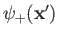, specifies the probability density of observing the particle at positionwith spin angular momentum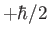in the-direction. The second,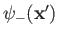, specifies the probability density of observing the particle at positionwith spin angular momentum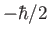in the-direction. In the Pauli scheme, these wavefunctions are combined into a spinor-wavefunction,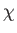, which is simply the column vector of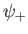and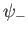. In general, the Hamiltonian is a function of the position, momentum, and spin operators. Adopting the Schrödinger representation, and the Pauli scheme, the energy eigenvalue problem reduces to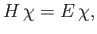(5.108)

whereis a spinor-wavefunction (i.e., a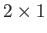matrix of wavefunctions) andis a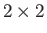matrix partial differential operator. [See Equation (5.92).] The previous spinor equation can always be written out explicitly as two coupled partial differential equations forand.

Suppose that the Hamiltonian has no dependence on the spin operators. In this case, the Hamiltonian is represented as diagonalmatrix partial differential operator in the Schrödinger/Pauli scheme. [See Equation (5.91).] In other words, the partial differential equation fordecouples from that for. In fact, both equations have the same form, so there is really only one differential equation. In this situation, the most general solution to Equation (5.108) can be written in the factorized form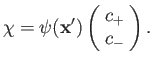(5.109)

Here,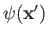is determined by the solution of the differential equation, and the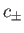are arbitrary complex numbers. The physical significance of the previous expression is clear. The Hamiltonian determines the relative probabilities of finding the particle at various different positions, but the direction of its spin angular momentum remains undetermined.

Suppose that the Hamiltonian depends only on the spin operators. In this case, the Hamiltonian is represented as amatrix of complex numbers in the Schrödinger/Pauli scheme [see Equation (5.74)], and the spinor eigenvalue equation (5.108) reduces to a straightforward matrix eigenvalue problem. The most general solution can again be written in the factorized form(5.110)

Here, the ratio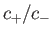is determined by the matrix eigenvalue problem, and the wavefunctionis arbitrary. Clearly, the Hamiltonian determines the direction of the particle's spin angular momentum, but leaves its position undetermined.

In general, of course, the Hamiltonian is a function of both position and spin operators. In this case, it is not possible to factorize the spinor-wavefunction as in Equations (5.109) and (5.110). In other words, a general Hamiltonian causes the direction of the particle's spin angular momentum to vary with position in some specified manner. This can only be represented as a spinor-wavefunction involving different wavefunctions,and.Next: Spin Greater Than One-Half Up: Spin Angular Momentum Previous: Spinor Rotation Matrices
Richard Fitzpatrick 2016-01-22